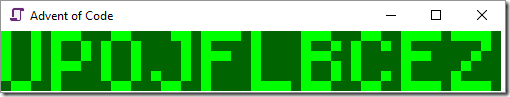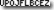Today’s Advent of Code challenge brought back memories of the day 6 puzzle from last year. In one sense, it was a typical Advent of Code challenge – parse the input into a sequence of instructions, work out how to apply each instruction, and then apply all the instructions on the input data.

The parsing could quite easily have been done with a bit of string splitting, but last year, but I really have been meaning to start making more use of “Active Patterns” in F#, a technique Sehnsucht put me onto last year.

And so I decided that the regular expression matching and int parsing would be done with active patterns. Here’s how I used them to parse instructions into an Instruction discriminated union:

``````open System.Text.RegularExpressions

type Instruction = Rect of int*int | RotateRow of int*int | RotateCol of int*int

let (|Matches|) pattern =
let rgx = Regex (pattern, RegexOptions.Compiled)
fun input -> [for m in rgx.Matches input do for g in m.Groups -> g.Value]

let (|Int|_|) str = match System.Int32.TryParse str with true, value -> Some value | _ -> None

let parseInstruction = function
| Matches @"rect (\d+)x(\d+)" [_; Int width; Int height] -> Rect(width,height)
| Matches @"rotate row y\=(\d+) by (\d+)" [_; Int row; Int steps] -> RotateRow(row,steps)
| Matches @"rotate column x\=(\d+) by (\d+)" [_; Int row; Int steps] -> RotateCol(row,steps)
| x -> failwith ("parseError " + x)

let instructions = System.IO.File.ReadAllLines (__SOURCE_DIRECTORY__ + "\\input.txt") |> Array.map parseInstruction
``````

To keep track of the state of each light, there were a lot of possible data structures. I opted simply for a 2D array of integers (could have been booleans, but integers allowed me to count lights on by summing the array). I would then create three functions to apply each of the instructions to the array.

Now this problem could be perfectly easily solved by mutating the array in place, although rotating a row or column is a bit of a pain to do in place. So I decided to make more “functional” instruction appliers by making them return a new 2D array with the relevant changes:

``````let rect (width,height) (state:int[,]) =
let out = Array2D.copy state
for w in 0..width-1 do
for h in 0..height-1 do
out.[h,w] <- 1
out

let rotateRow (row,steps) (state:int[,]) =
let out = Array2D.copy state
let rowLength = Array2D.length2 state
for w in 0..rowLength-1 do
out.[row,(w+steps)%rowLength] <- state.[row,w]
out

let rotateCol (col,steps) (state:int[,]) =
let out = Array2D.copy state
let colLength = Array2D.length1 state
for w in 0..colLength-1 do
out.[(w+steps)%colLength,col] <- state.[w,col]
out
``````

There is a performance cost to copying the arrays, but for the size of array and number of instructions it’s not a big deal.

Now we can make a folder function that applies each instruction, and use `Array.fold` to apply it onto a starting empty array. Then we use the `Seq.cast` trick to flatten the 2D array into a sequence of all elements in all rows, and sum them.

``````let applyInst (state:int[,]) = function
| Rect(a,b) -> rect (a,b) state
| RotateRow(a,b) -> rotateRow (a,b) state
| RotateCol(a,b) -> rotateCol (a,b) state

instructions
|> Array.fold applyInst (Array2D.create 6 50 0)
|> Seq.cast<int>
|> Seq.sum
|> printfn "Part a: %d"
``````

Now the second part of the puzzle was great. You simply had to visualize your LCD display to read off the code shown by the lights. Obviously there were a number of ways to do this, including by just printing to the console.

But I did two simple visualizations. First, I drew it using WPF. I created a canvas and added a rectangle for each on pixel, and used a render transform to increase the size:

``````#if INTERACTIVE
#r "PresentationCore.dll"
#r "PresentationFramework.dll"
#r "System.Xaml.dll"
#r "UIAutomationTypes.dll"
#r "WindowsBase.dll"
#endif

open System.Windows
open System.Windows.Media
open System.Windows.Media.Imaging
open System.Windows.Shapes
open System.Windows.Controls

let drawState (state:int[,]) scale =

let width = Array2D.length2 state
let height = Array2D.length1 state
let canvas = new Canvas(Background=Brushes.DarkGreen,
RenderTransform=ScaleTransform(ScaleX = scale, ScaleY = scale),
Width = (float width),
Height = (float height),
HorizontalAlignment = HorizontalAlignment.Left,
VerticalAlignment = VerticalAlignment.Top
)
for x in {0..width-1} do
for y in {0..height-1} do
if state.[y,x] = 1 then
let p = Rectangle(Width=1.0,Height=1.0,Fill=Brushes.Lime)
Canvas.SetLeft(p, float x)
Canvas.SetTop(p, float y)
printfn "%f %f" canvas.Width canvas.Height
canvas

let scaleFactor = 10.0
let canvas = drawState endState scaleFactor
let window = Window(Title ="Advent of Code", Content=canvas,
Width = 20.0 +  canvas.Width * scaleFactor, Height = 40.0 + canvas.Height * scaleFactor)
window.ShowDialog()
``````

This gives us a visualization like this:Obviously if we wanted to be really fancy we could use a timer, and animate the full sequence as we process each instruction. There were some fantastic visualizations from the community on Reddit.

One thing I didn’t like about my solution was that the code wasn’t calculated programmatically. I did wonder whether I could use an OCR library, or even send the image off to Microsoft Cognitive Services to OCR it. I think it would work, although the code I wrote to make a bitmap made an image that cognitive services rejected for being too small. Here’s the code to turn the puzzle output into a bitmap with WinForms though:

``````open System.Windows.Forms
open System.Drawing

let drawState (state:int[,]) =
let width =Array2D.length2 state
let height = Array2D.length1 state
let bm = new Bitmap (width, height)
for x in {0..width-1} do
for y in {0..height-1} do
bm.SetPixel(x,y, if state.[y,x] = 1 then Color.Black else Color.White)
bm

let image  = drawState endState
image.Save("output.bmp")
``````

This creates a tiny bitmap containing our answerAs always, there’s lots of ways my solution could be improved on, but the main thing is that I’m learning a few new techniques as I go.